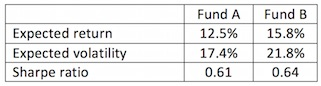### CFA Practice Question

There are 255 practice questions for this topic.

### CFA Practice Question

The risk-free rate is 1.8%. You have a choice of investing in two funds:You want to accept a maximum volatility of 17.4% only. Assume you cannot borrow. What is the maximum expected return you can possibly get?
A. 12.5%
B. 12.64%
C. 12.78%
Explanation: If you invest 80% in Fund B and 20% in cash, the expected volatility of your portfolio will be 21.8% x 80% = 17.4%. Your expected return will be 15.8% x 80% + 1.8% x 20% = 12.64%, which is higher than investing in Fund A alone (with the same risk).

User Comment
ange yes they do. remember to deduct 1.8% from the expected returns.
tr4nceur how do the numbers add up
tr4nceur wait nevermind - return from investing is cash is known with certainty hence needs no expectation
truss88 they should clarify the question as it is possible for the correlation to be -1 between A and B, in which case investing .7962 in B and the rest in A gets you a return of 15.1%.
gkbarr88 Why not invest 20% into fund A instead of cash?
gkbarr88 Bunk question. Sharpe ratios don't tie out either.
stefalf Investing 80% in Fund B and 20% in the risk free will indeed give you the 12.64% return. It will however also leave you with a volatility of 17.44% which is higher than the 17.4% stated.

The solution should be investing 17.4/21.8= 0.7982 in Fund B, which is 0.7982*15.8 = 12.61, and investing the remaining 0.2018 in the risk-free asset (not Fund A as it will increase your volatility), which is 0.2018*1.8=0.3633.

Total return: 12.6110 + 0.3633 = 12.97
JNW1980 I think the problem solution assumes you just leave the excess 20% in cash wheras if you invested in the RF rate then you would get the 12.97%

You can figure this out by reworking the formulas and actually solving for the RF rate using the sharpe ratios.

.64*.218 = Ra - Rf Therefore Rf = .158 - .64*.218 = 1.848 (close to the 1.8% given). Reworking the sharpe equation with the required 17.4% expected volatility in the denominator will yield you either 12.97 or 12.98 expected return assuming you invested the remainder in the Rf rate